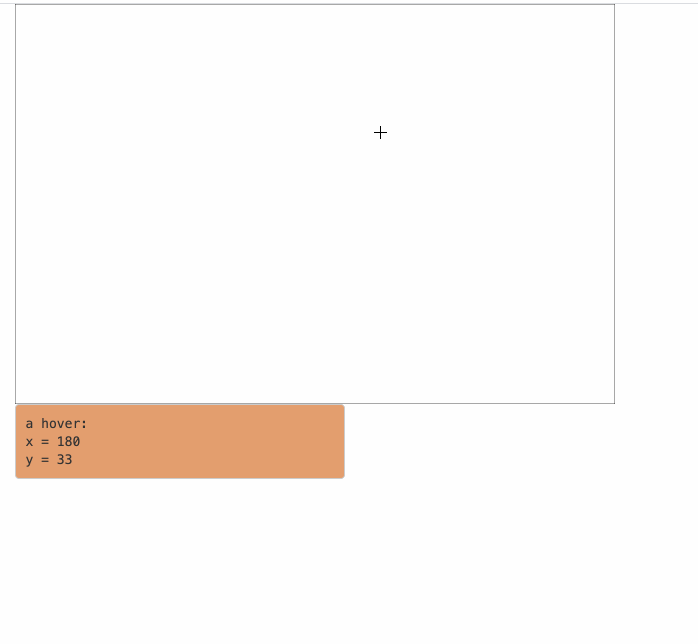# Differentiate brush and hover event in Shiny

In my last post I described how I distinguish click and brush event in plotOutput in Shiny. In this post I try to solve another problem which is to differentiate hover and brush event.

Similar as click, brush also intializes a hover event. To distinguish hover and brush, I didn’t use the default hover in plotOutput. Here I implement my own one. The implementation contains two parts:

1. the hover event is invoked after the mouse position keeps unchanged for a peroid of time, e.g. 300ms.
2. the brush action starts with a hover action, then instantly, a div which corresponds to the brush is created.

For part 1, we need a mouse event which stops at a certain position for a period of time. Here I use mousestop.js. And for the part 2, we just simply test whether the brush div exists or not.

In the following example code, since mousestop does not support a function with event as argument, I additionally use mousemove to catch the mouse position and assign in mousestop.

library(circlize)
library(GetoptLong)
library(grid)
library(shiny)
library(glue)

ui = fluidPage(
plotOutput("plot", width = 600, height = 400,
brush = "brush"),
tags$script(HTML(" var relX = -1; var relY = -1;$('#plot').mousemove(function(e) {
var parentOffset = $(this).offset(); relX = e.pageX - parentOffset.left; relY = e.pageY - parentOffset.top; }).mousestop(function() { if($('#plot_brush').length == 0) {
Shiny.setInputValue('x', relX);
Shiny.setInputValue('y', relY);
Shiny.setInputValue('action', Math.random());
}
});
")),
fluidRow(
column(3, htmlOutput("output1")),
column(3, htmlOutput("output2"))
)
)

server = function(input, output, session) {
output$plot = renderPlot({ grid.newpage() grid.rect() }) observeEvent(input$action, {
output$output1 = renderText({ isolate(glue(" <pre style='background-color:{rand_color(1)}'> a hover: x = {input$x}
y = {input$y}</pre>")) }) }) observeEvent(input$brush, {
output$output2 = renderText({ isolate(glue(" <pre style='background-color:{rand_color(1)}'> a brush: input$brush$coords_css$xmin = {input$brush$coords_css$xmin} input$brush$coords_css$ymin = {input$brush$coords_css$ymin} input$brush$coords_css$xmax = {input$brush$coords_css$xmax} input$brush$coords_css$ymax = {input$brush$coords_css\$ymax}</pre>"))
})
})
}

shinyApp(ui, server)

The demo is in the following figure: